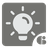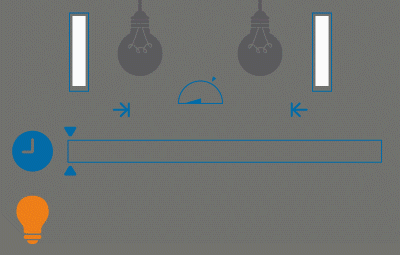Lightbulb More
Simple+
English RU

The complication in the 6th mission of the series is that now there might be needed more than one light bulb to illuminate a room. And this is the 5th argument of the function - how many light bulbs are needed to illuminate the room.

For example, if you need 3 bulbs to illuminate a room, then we don’t count the time when there were only 2 bulbs or only one. If the last argument of the function is not passed, then one light bulb is enough to illuminate the room.

The task is still the same - to find out how long the room was lit (in this task, we can say - sufficiently lit).Input: Five arguments and only the first one is required. The first one (els) is a list of datetime objects (instead of datetime object there can be a tuple of two datetime and int), the second (start_watching) and the third ones (end_watching) are the datetime objects. The forth argument (operating) - timedelta object - how long the lamp can work. The 5th argument is a positive non-zero int.

Output: A number of seconds as an integer.

Example:

```sum_light([
(datetime(2015, 1, 12, 10, 0, 10), 3),
datetime(2015, 1, 12, 10, 0, 20),
(datetime(2015, 1, 12, 10, 0, 30), 3),
(datetime(2015, 1, 12, 10, 0, 30), 2),
datetime(2015, 1, 12, 10, 0, 40),
(datetime(2015, 1, 12, 10, 0, 50), 2),
], req=2) == 20

sum_light([
(datetime(2015, 1, 12, 10, 0, 10), 3),
datetime(2015, 1, 12, 10, 0, 20),
(datetime(2015, 1, 12, 10, 0, 30), 3),
(datetime(2015, 1, 12, 10, 0, 30), 2),
datetime(2015, 1, 12, 10, 0, 40),
(datetime(2015, 1, 12, 10, 0, 50), 2),
], req=3) == 0

sum_light([
(datetime(2015, 1, 12, 10, 0, 10), 3),
datetime(2015, 1, 12, 10, 0, 20),
(datetime(2015, 1, 12, 10, 0, 30), 2),
(datetime(2015, 1, 12, 10, 0, 50), 3),
datetime(2015, 1, 12, 10, 0, 40),
(datetime(2015, 1, 12, 10, 0, 50), 2),
], req=3) == 10
```

Precondition:

• The array of pressing the button is always sorted in ascending order.
• The array of pressing the button has no repeated elements.
• The minimum possible date is 1970-01-01
• The maximum possible date is 9999-12-31
• req arguments is positive and non-zero